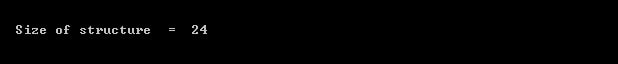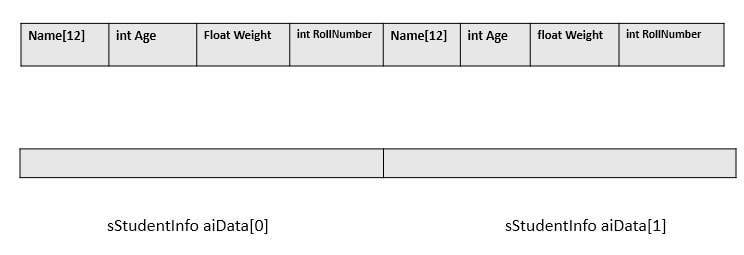# How to find the size of structure in c without using sizeof operator?In C language, sizeof() operator is used to calculate the size of any variables, pointers or data types, data types could be pre-defined or user-defined.Using the sizeof() operator we can calculate the size of the structure straightforward to pass it as a parameter.

But here I am interested in calculating the size of structure in c without using the sizeof() operator. Calculating the size of a structure in c without using the sizeof() operator seems to be difficult but with the help of pointers, we can do it easily. It is also an important interview question which is generally asked by the interviewer to check the understanding of pointer.

In this article, I will describe few methods to calculate the size of the structure in c without using the sizeof operator.But it is my recommendation to use the sizeof() operator to calculate the size of the structure in a program whenever you need to calculate the size of the structure.

Here we need to use some techniques to calculate the size of the structure in c.I am describing this technique, using few examples.

Note: Structure padding also affects the size of the structure in C.

### Example  1

• First, create the structure.
• After creating the structure, create an array of structure, Here RamInfo.
• Create a pointer to structure and assign the address of the array.

### Example 2

When we increment the pointer then pointer increase a block of memory (block of memory depends on pointer data type). So here we will use this technique to calculate the size of a structure.

See this link: pointer arithmetic

• First, create the structure.
• Create a pointer to structure and assign the NULL pointer.
• Increment the pointer to 1.##### If you want to learn more about the c language, here 10 Free days  C video course for you.### Example 3

We can also calculate the size of the structure using the pointer subtraction. In the previous article “all about the pointer“, we have read that using the pointer subtraction we can calculate the number of bytes between the two pointers.

• First, create the structure.
• create an array of structure, Here aiData.
• Create pointers to the structure and assign the address of the first and second element of the array.
• Subtract the pointers to get the size of the structure in c.1.Mark Odell says:
2.Amlendra says:
3.sowmya says: